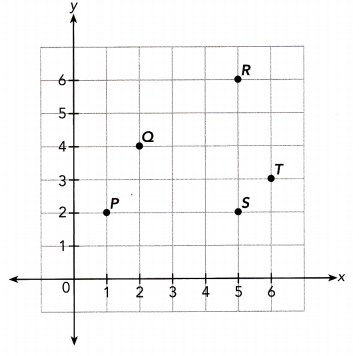# Math in Focus Grade 7 Chapter 5 Answer Key Direct and Inverse Proportion

Practice the problems of Math in Focus Grade 7 Workbook Answer Key Chapter 5 Direct and Inverse Proportion to score better marks in the exam.

## Math in Focus Grade 7 Course 2 A Chapter 5 Answer Key Direct and Inverse Proportion

### Math in Focus Grade 7 Chapter 5 Quick Check Answer Key

Write a ratio to compare quantities.
A store sells 60 headphones, 45 sets of earbuds, and 80 speakers. Write a ratio in simplest form to compare each of the following.

Question 1.
The number of speakers to the number of sets of earbuds.
Ratio of earbuds and speakers = 9:16.

Explanation:
Number of headphones a store sells = 60.
Number of earbuds a store sells = 45.
Number of speakers a store sells = 80.
Ratio of earbuds and speakers = Number of earbuds a store sells: Number of speakers a store sells
= 45:80
= 9:16.

Question 2.
The number of headphones to the number of speakers.
Ratio of headphones and speakers = 3:4.

Explanation:
Number of headphones a store sells = 60.
Number of speakers a store sells = 80.
Ratio of headphones and speakers = Number of headphones a store sells: Number of speakers a store sells
= 60:80
= 6:8
= 3:4.

Tell whether each pair of ratios are equivalent.
Question 3.
9 : 11 and 18 : 22
9 : 11 and 18 : 22 are equivalent pairs of ratios.

Explanation:
9:11
18:22 = 9:11.

Question 4.
$$\frac{1}{33}$$ and $$\frac{33}{1}$$
$$\frac{1}{33}$$ and $$\frac{33}{1}$$ are not equivalent pairs of ratios.

Explanation:
$$\frac{1}{33}$$ = 1:33.
$$\frac{33}{1}$$ = 33:1.

Question 5.
3 to 6 and 9 to 18
3 to 6 and 9 to 18 are equivalent pairs of ratios.

Explanation:
3 to 6 = 3:6 = 1:2.
9 to 18 = 9:18 = 1:2.

Tell whether each ratio is in simplest form. Then write two ratios that are equivalent to the given ratio.
Question 6.
4 : 5
4:5 ratio is in simplest form.

Explanation:
4:5
16:20 = 4:5.
12:15 = 4:5.

Question 7.
$$\frac{15}{100}$$
$$\frac{15}{100}$$ ratio is not in simplest form.

Explanation:
$$\frac{15}{100}$$ = 15:100 = 3:20.

Question 8.
7 to 14
7 to 14 ratio is not in simplest form.

Explanation:
7 to 14 = 7:14 = 1:2.

Find the unit rate.
Question 9.
The winner of the first Tour de France bicycle race in 1903 was Maurice Garin. It took him over 94 hours to complete 2,428 kilometers. Find his approximate average speed. Round your answer to the nearest whole number.
Average speed of Maurice Garin = 30 kilometer/hour.

Explanation:
Number of kilometers Maurice Garin completes = 2,428.
Number of hours Maurice Garin takes = 94.
Average speed of Maurice Garin = Number of kilometers Maurice Garin completes ÷ Number of hours Maurice Garin takes
= 2,428 ÷ 94
= 25.83 kilometer/hour.
Nearest whole number of Average speed of Maurice Garin = 30 kilometer/hour.

Find and compare unit rates.
The cost of a food item at two different stores is shown. Find the unit price at each store and tell where the item costs less.

Question 10.
Store A: $3.20 for 16 oz of walnuts. Store B:$2.30 for 10 oz of walnuts.
Unit price at store A = $0.2. Unit price at store B =$2.3.
Store A items costs less.

Explanation:
Store A: $3.20 for 16 oz of walnuts. Store B:$2.30 for 10 oz of walnuts.
Unit price at store A = Cost of walnuts ÷ Number of walnuts
= $3.20 ÷ 16 =$0.2.
Unit price at store B = Cost of walnuts ÷ Number of walnuts
= $2.30 ÷ 10 =$2.3.

Question 11.
Store C: $2.13 for 3 Ib of potatoes. Store D:$3.35 for 5 Ib of potatoes.
Unit price at store C = $0.71. Unit price at store D =$0.67.
Store D items costs less.

Explanation:
Store C: $2.13 for 3 Ib of potatoes. Store D:$3.35 for 5 Ib of potatoes.
Unit price at store C = Cost of potatoes ÷ Number of potatoes
= $2.13 ÷ 3 =$0.71.
Unit price at store D = Cost of potatoes ÷ Number of potatoes
= $3.35 ÷ 5 =$0.67.

Use the coordinate plane below.Question 12.
Give the coordinates of points P, Q, R. S, and T.
coordinates of point of  P = (1,2)
coordinates of point of Q = (2,4)
coordinates of point of R = (5,6)
coordinates of point of  S = (5,2)
coordinates of point of T = (6,3)

Explanation:
coordinates of point of  P = (1,2)
coordinates of point of Q = (2,4)
coordinates of point of R = (5,6)
coordinates of point of  S = (5,2)
coordinates of point of T = (6,3)

Solve word problems involving percent.
Question 13.
45% of the beads in a box are blue. If there are 36 blue beads in the box, how many beads are there altogether?
Total number of beads altogether = 80.

Explanation:
45% of the beads in a box are blue.
Number of blue beads in the box = 36.
Tabitha bought an antique model car priced at $72. She also had to pay 5% sales tax. What was the total amount she paid? Answer: Total amount she paid =$75.6.
Cost of an antique model car Tabitha bought = $72. Sales tax an antique model car Tabitha bought = 5% Total amount she paid = Cost of an antique model car Tabitha bought + (Sales tax an antique model car Tabitha bought × Cost of an antique model car Tabitha bought) =$72 + (5% × $72) =$72 + (1­ ÷ 20 × $72) =$72 + ($3.6) =$72 + $3.6 =$75.6.